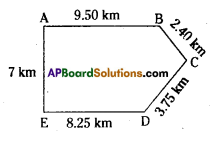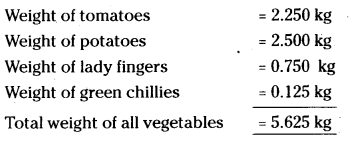AP Board 7th Class Maths Solutions Chapter 2 Fractions, Decimals and Rational Numbers Ex 5

AP State Syllabus AP Board 7th Class Maths Solutions Chapter 2 Fractions, Decimals and Rational Numbers Ex 5 Textbook Questions and Answers.

AP State Syllabus 7th Class Maths Solutions 2nd Lesson Fractions, Decimals and Rational Numbers Exercise 5Question 1.
Which one is greater?
(i) 0.7 or 0.07
(ii) 7 or 8.5
(iii) 1.47or 1.51
(iv) 6 or 0.66
1 cm = 10 mm
1 m = 100cm
1 km = 1000m
1kg =1000gm
Solution:
i) 0.7 or 0.07 = 0.7 is greater
ii) 7 or 8.5= 8.5 is greater
iii) 1.47 or 1.50 = 1.50 is greater
iv) 6 or 0.66 = 6 is greaterQuestion 2.
Express the following as rupees using decimals.
(i) 9 paise
(ii) 77 rupees 7 paise
(iii) 235 paise
Solution:
(i) 9 paise = $$\frac { 9 }{ 100 }$$ = ₹ 0.09
(ii) 77 rupees 7 paise = 77 rupees $$\frac { 7 }{ 100 }$$ rupees = ₹ 77.07
(iii) 235 paise = ₹ $$\frac{235}{100}$$ = ₹ 2.35

Question 3.
(i) Express 10 cm in metre and kilometre.
(ii) Express 45 mm in centimeter, meter and kilometer.
Solution:
i) 10cm = $$\frac{10}{100}$$ m = 0.1 m
1o cm = $$\frac{10}{100 \times 1000}$$ km = 0.0001 km

ii) 45 mm = $$\frac{45}{10}$$ cm = 4.5 cm
= $$\frac{4.5}{100}$$ m = 0.045 m
= $$\frac{0.045}{1000}$$ km = 0.000045 km

Question 4.
Express the following in kilograms.
(i) 190g
(ii) 247g
(iii) 44kg 80gm
Solution:
(i) 190g = $$\frac{190}{1000}$$ = 0.190 kg
(ii) 247g = $$\frac{247}{1000}$$ kg = 0.247 kg
(iii) 44kg 80gm = 44 kg $$\frac{80}{1000}$$ kg = 44.080kgQuestion 5.
Write the following decimal numbers in expanded form.
(i) 55.5
(ii) 5.55
(iii) 303.03
(iv) 30.303
(v) 1234.56
Solution:
(i) 55.5 = 10 × 5 + 1 × 5 × $$\frac{1}{10}$$ × 5 = 50 + 5 + $$\frac{5}{10}$$
(ii) 5.55 = 1 × 5 + $$\frac{1}{10}$$ × 5 + $$\frac{1}{100}$$ × 5 = 5 + $$\frac{5}{10}+\frac{5}{100}$$
(iii) 303.03 = 100 × 3 + 1 × 3 + $$\frac{1}{100}$$ × 3 = 300 + 3 + $$\frac{3}{100}$$
(iv) 30.303 = 10 × 3 + $$\frac{1}{10}$$ x 3 + $$\frac{1}{1000}$$ x 3 = 30 + $$\frac{3}{10}+\frac{3}{1000}$$
(v) 1234.56 = 1000 × 1 + 100 × 2 + 10 × 3 + 1 × 4 + $$\frac{1}{10}$$ × 5 + $$\frac{1}{100}$$ × 6 = 1000 + 200 + 30 + 4 + $$\frac{5}{10}+\frac{6}{100}$$Question 6.
Write the place value of 3 in the following decimal numbers.
(i) 3.46
(ii) 32.46
(iii) 7.43
(iv) 90.30
(v) 794.037
Solution:
i) 3.46 – place value of 3 in 3.46 is 3 × 1 =-3
ii) 32.46- place value of 3 in 32.46 is 3 × 10 = 30
iii) 7.43- place value of 3 in 7.43 is 3 × $$\frac{1}{100}$$ = 0.03
iv) 90.30- place value of 3 in 90.30 is 3 × $$\frac{1}{10}$$ = 0.3
v) 794.037 – place value of 3 in 794.037 is 3 × $$\frac{1}{100}$$ = 0.03

Question 7.
Aruna and Radha start their journey from two different places. A and E. Aruna chose the path from A to B then to km C, while Radha chose the path from E to D then to C. Find who traveled more and by how much?
Solution:
Distance covered by Aruna = AB + BC
= 9.50+2.40 = 11.90km
Distance covered by Rad ha = ED + DC
= 8.25 + 3.75 = 12 km
Radha travelled more by (12.00 – 11.90) = 0.10 km.Question 8.
Upendra went to the market to buy vegetables. He brought 2 kg 250 gm tomatoes, 2 kg 500gm potatoes, 750gm lady fingers and 125 gm green chillies. How much weight did Upendra cany back to his house?Upendra carried back a total weight of 5.625 kg